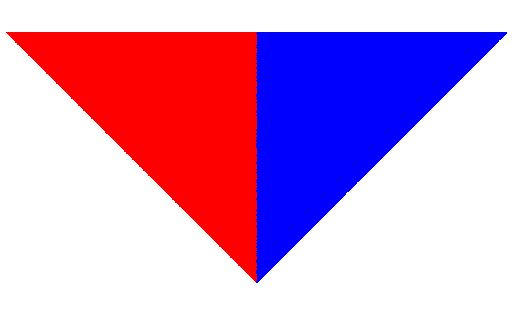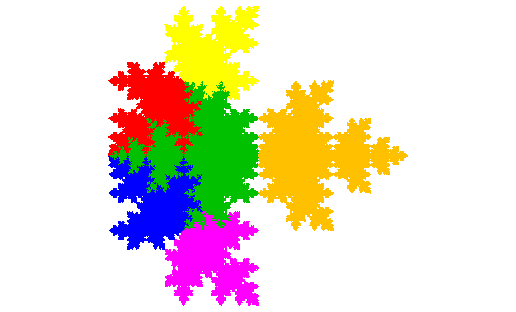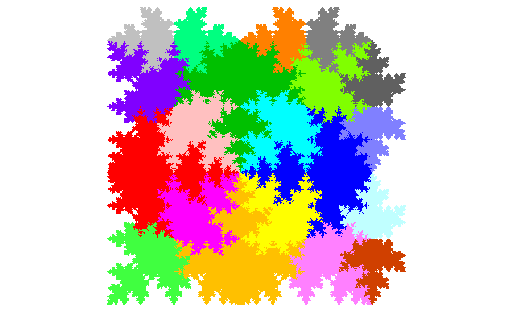# Mapleleaf TileThe Cesaro triangle is a rep-2 tile, which can serve as a base for further investigation of attractors of IFS systems. The autocomposition technique can be applied to this to give a rep-4 implementation of the same right isosceles triangle. (I believe that this corresponds to a space filling curve attributed to Polya.)The partial post autocomposition (meta-figure) technique can be applied to this rep-4 right isosceles triangle to give the figure to the left. Note that this figure has a straight line boundary. In the past reflection of some elements in such boundaries (e.g. in deriving the metatrapezoidal snowflake from the star metatrapezium) has been productive.And sure enough reflecting the red and yellow elements in this boundary gives us a d2-symmetric fractal.The grouped element technique can then be used to create the mapleleaf tile. (As the preceding fractal consists of two copies of the mapleleaf tile we can call it the double mapleleaf.) The mapleleaf tile tiles the plane. The unit cell contains 4 full size copies, 8 half size copies and 8 quarter size copies.We start by placing the 4 full size copies in a ring. This leaves large voids ...... which can be filled by 4 half sized copies to create a compact figure. However this figure does not appear to tile the plane.Adding 4 more half sized figures creates a figure which touches the boundaries of its enclosing square at an infinite number of points (as does the preceding, so it's less obvious as it doesn't even approach the corners, whereas this one touches the corners). However this figure also doesn't tile the plane, gaps being left along the edges of the square.But 4 quarter sized figures can be added to one edge ..and 4 more to another edge, to create the unit cell for the tiling of the plane by the mapleleaf tile. Note that the double mapleleaf also tiles the plane, with half the number of copies in the unit cell, as can be seen by pairing elements of the unit cell for the mapleleaf tile. Similarly the figure preceeding the double mapleleaf, also being a half of a double mapleleaf, also tiles the plane, with the same number and sizes of copies as the mapleleaf tile.

Source: independent discovery.

data file mapletile.xil; images generated at 3x3 per screen at 1280x1024